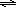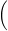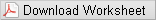# Lab Investigation 6 - How Well Can a Buffer Resist pH Change?

## Guiding Question

How well can a buffer resist pH change?

## Introduction

In dilute aqueous solutions, weak acids are slightly dissociated. They produce a small concentration of hydronium ion (H3O+) and an equal concentration of the conjugate base of the acid. Such dissociation reactions are equilibria, and equilibrium mathematics can be used to calculate concentrations of the species present in solution. Consider formic acid (CH2O2); its dissociation constant (Ka) is
1.7 × 10−4.
Incidentally, formic acid is what red ants inject when they bite. The concentration of H3O+ present in a 0.010-M solution of formic acid can be calculated from the equilibrium expression and a reaction table.
( 1 )
HCOOH(aq)   +   H2O(l)H3O+(aq)   +   HCOO(aq)

 initial 0.010 0 0 Δ –x +x +x equilibrium 0.010 – x x x
( 2 )
Ka = 1.7 × 10−4 =
 [H3O+][HCOO−] [HCOOH]
=
 x2 0.010 − x

Solving for x, one finds that the solution is 0.0012 M in H3O+ and HCOO. Expressing [H3O+] as a pH,
( 3 )
pH = −log [H3O+] = −log(0.0012) = 2.92.
Although a weak acid dissociates only slightly in water, the soluble salt of a weak acid (for example, sodium formate) is a strong electrolyte and dissociates completely.
( 4 )
HCOONa(s) + H2O(l) → Na+(aq) + HCOO(aq
( 5 )
pH = pKa + log[base] [acid]or pH = pKa + logmoles of base moles of acidThe derivation of this equation follows from the general dissociation equilibrium expression for a weak acid, and includes the assumption that [H3O+] is small relative to [HA].
( 6 )
HA + H2OH3O+ + A

( 7 )
Ka =
 [H3O+][A−] [HA]

Solving for [H3O+] gives:
( 8 )
[H3O+] =
 Ka × [HA] [A−]
Taking the negative logarithm of both sides puts the equation in terms of pH:
( 9 )
pH = −log [H3O+] = −log Ka − log([HA]/[A]).
By definition,
pKa = −log Ka and −log([HA]/[A]) = log([A]/[HA]).
Substituting these terms into equation 9 :
( 10 )
pH = pKa + log([A]/[HA]).
Equation 5 shows that pH can be found using either concentrations of acid and base, or the number of moles of each. This follows from the fact that the volume term is the same for the acid and its conjugate base, and cancels in the calculation.In the pre-lab exercise, you will prepare various acetate buffer solutions by the direct method and measure the pH of each solution. In the direct method, the conjugate acid and base are added together in solution to get the desired base to acid ratio. For example, acetic acid and sodium acetate will be combined in solution. In the buffer investigation you will attempt to prepare an acetate buffer of pH 5.00 and determine the buffer capacity with respect to strong acid and strong base.

## Goals

As you complete this investigation you will:
• 1
Prepare a buffer with a pH of ~5.00.
• 2
Verify the pH of the buffer.
• 3
Determine the buffer capacity with regard to strong acid and strong base.

## Materials Available For Use

• Pipettor and tips
• Beakers
• Buret
• Funnel
• Analytical balances
• Vernier LabPro system
• pH probe
• Probe calibration solutions
• 6.0 and 1.0 M acetic acid (HC2H3O2)
• Sodium acetate (NaC2H3O2)
• 0.50 and 0.050 M NaOH(aq)
• 0.50 and 0.050 M HCl(aq)

## Safety Precautions

Caution:
Acetic acid, HCl, and NaOH are corrosive. They can attack the skin and cause permanent damage to the eyes. If one of these solutions splashes into your eyes, use the eyewash station immediately. Hold your eyes open and flush with water. If contact with skin or clothing occurs, flush the affected area with water. Have your lab partner notify your instructor about the spill.

## Waste Disposal

All solutions can be flushed down the sink with water.

## Getting Started

• 1
What reagents will you use to obtain desired pH?
• 2
What amounts of reagents will you use to produce the buffer?
• 3
Review titration techniques in preparation for determining the buffer capacity.

## Procedure

1
You need to prepare 100 mL of the buffer solution using the appropriate volume of 1.0 M acetic acid and the appropriate mass of sodium acetate. You will want the concentrations relatively dilute, so make the acid concentration 0.10 M or 0.010 M.
2
Check the pH of your buffer. It should be within ±0.2 units.
3
Buffer capacity is defined as the number of moles of strong base or strong acid required to cause a one-unit change in pH of a buffer solution.
• a
Determine the buffer capacity for strong acid by titrating 25.0 mL of your buffer with 0.50 M HCl for the 0.1 M buffer or 0.050 M HCl for the 0.01 M buffer until the pH decreases by 1 unit. Report the capacity as moles acid per L buffer.
• b
Determine the buffer capacity for strong base by titrating 25.0 mL of your buffer with 0.50 M NaOH for the 0.1 M buffer or 0.050 M NaOH for the 0.01 M buffer until the pH increases by 1 unit. Report the capacity as moles base per L buffer.

## Interactive Poster Session

Once your group has completed your work, prepare a whiteboard that you can use to share and justify your ideas. See the handout provided for details on this process.

## Report

Once you have completed your research, you will need to prepare an investigation report that consists of three sections. This report may require more than 2 pages with data tables. This report must be typed and any diagrams, figures, or tables should be embedded into the document.
• Section 1: What concept were you investigating, and how does it relate to the guiding question? How well can a buffer resist pH change? Define and explain relevant terms, buffer, pH, and buffer capacity. See the getting started questions for suggestions as well as the introduction.
• Section 2: Outline the calculations used to determine the amounts of materials you used in the buffer solution. Describe the procedure you used to prepare the buffer and to measure the buffer capacity.
• Section 3: What is your argument? Present data for pH and buffer capacity. Show a calculation for prediction of buffer capacity. How did experimental pH and the buffer capacity of your prepared buffer compare to the calculated values? What would cause variations? What range of pH could you buffer with your proposed system? How did your system compare with other groups, particularly those that worked with a different buffer system?
• This third section is where you not only present your data, but use the values you obtain as evidence in your reasoning. Statements like, "see data table for values" are not acceptable!
Remember: An argument is not just an answer to the question. It is a claim or conclusion supported by evidence with a rationale for why the evidence supports the claim or conclusion.